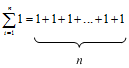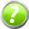x2
Introduction
( )
Types of Arrangements
=
Two More Methods
=
Some Applications

# Series and the Sigma Notation (Part 2)

Quick Navigation Video Lectures Exercises

# Example 7 - Writing a Series

Write $-2x^2+3x^3-4x^4$ using the Σ notation.

There are three things that stand out to me in this expression:

1. The exponents start at 1 and increase by 1 in each term. That makes me think there's going to be an $x^i$ in the formula.
2. The coefficients start at 1 and increase by 1 in each term. I can add that to the formula by putting an i in front of it: $ix^i$.
3. The signs alternate starting with a +. This is where it gets a little tricky. If I add $(-1)^i$ to the formula, that will make the signs alternate but starting with a - where our expression starts with a +. I can fix that by changing the i to i + 1: (-1)i + 1. Now, when i = 1 that part will be (-1)1 + 1 = (-1)2 = +1, when i = 2 it will be (-1)1 + 2 = (-1)3 = -1, etc.

That makes our formula $(-1)^{i+1} \cdot ix^i$. Because our terms start at 2 and end at 4, our final sum must be

$$\sum_{i=1}^{3}(-1)^{i+1} \cdot ix^i$$

# Example 8 - Writing a Series

Write the series $8x^5 + 16x^6 + 32x^7 + 64x^8$.

The first thing I notice here is that the coefficients are powers of 2:

$$2^3x^5 + 2^4x^6 + 2^5x^7 + 2^6x^8$$

What makes this a little tricky is that the exponents on the 2's and the x's are out of synch with each other. One starts at 3 where the other starts at 5. We can fix that by writing the bigger numbers this way:

$$2^3x^{3+2} + 2^4x^{4+2} + 2^5x^{5+2} + 2^6x^{6+2}$$

Now, the exponents of the 2 start at 3 and go to 6 and the exponents of the x's start at 3 + 2 and go to 6 + 2. That makes our formula $2^i x^{i+2}$ where i starts at 3 and goes to 6.

$$\sum_{i=3}^6 2^i x^{i+2}$$

Pay close attention to what was going on in Example 7. The technique of using i for one of the parts and i + (a number) is called using an "offset". Whenever you see that two parts of the series both have the "increase by 1" pattern but they don't start at the same value, that's when you should think about using this technique. Once you've identified this situation, here's how you create the formula:

1. The smaller of the two numbers will use i. This is the 2 in Example 7 because its exponents are smaller than the exponents on the x's.
2. The larger of the two numbers will use i + (a number) where (a number) is the difference between the two values. In Example 7, this was the exponents on the x's. The difference between the first two exponents was 5 - 3 = 2 so, for the x exponents, I used i + 2.

# Example 9 - Series Formulas

Show that $\sum_{i = 1}^n 1=n$.

With this question, I think it will be helpful to go back to using a table to write out the terms of the sum:

 i 1 1 1 2 1 . . . n n

(Remember that the dots, . . ., indicate that the pattern you see in the other numbers continues. In this case, it means that there's a 1 in every column.)

What makes this series a little unusual is that there isn't an i in the formula. That's a little strange but there's nothing wrong with it. It just means that each term of the series is equal to 1, as you can see in the table. That means thatBut the sum of n 1's is just n which means that

$$\sum_{i = 1}^n 1=n$$

# Example 10 - Series Formulas

Write out the series $\sum_{i = 1}^n x^i + \sum_{i = 1}^n y^i$.

With an expression like this, it can help to write out the terms.

$$(x^{1} + x^{2} + ... + x^{n}) + (y^{1} + y^{2} + ... + y^{n})$$

Now, suppose I rearrange the terms so that the ones with the same exponents are together. $$(x^{1} + y^{1}) + (x^{2} + y^{2}) + ... (x^{n} + y^{n})$$

Now, notice how the exponents follow our "increasing by 1" pattern. They go from 1 in $x^{1} + y^{1}$ to 2 in $x^{2} + y^{2}$, etc. That makes me think that I can make a new formula with $x^{i}+y^{i}$ where i goes from 1 to n. That would make the series

$$\sum_{i = 1}^n (x^i + y^i)$$

# Exercises

### Exercises= show solution= get hint

l
1. $\sum_{i=0}^4 3i$
2. $\sum_{i=0}^4 x^{2i}$
3. $\sum_{i=1}^7 (i+1)^2$
4. $\sum_{i=0}^4 ix^{i-1}$
5. $\sum_{i=0}^4 (-1)^i x$
6. $\sum_{i=5}^6 \frac{i}{3}x$
7. $\sum_{i=1}^3 \frac{i}{x}$
8. $\sum_{i=2}^5 (x^{2i + i}$
9. $\sum_{i=2}^5 (-2)^i (ix+1)^2$
10. 1 + 2 + 3 + 4 + 5 + 6 + 7 + 8 + 9
11. $x^2+x^4+x^6+x^7$
12. $1 + \frac{1}{2} + \frac{1}{3} + \frac{1}{4} + \frac{1}{5}$
13. $1 + x + 2x^{2} + 6x^{3} + 24x^{4} + 120x^{5}$
14. $-x + 2x^{2} - 4x^{3} + 8x^{4}$
15. $-2x + 4x^{2} - 8x^{3} + 16x^{4}$
16. $-4x^{2} + 5x^{3} - 6x^{4} + 7x^{5}$
17. $\frac{x}{1} - \frac{x^2}{2} + \frac{x^3}{6} - \frac{x^4}{24} + \frac{x^5}{120}$

### Analysis

1. Explain why the series in example 4 is equal to $\sum_{i=1}^3 (2i)x^{i+1}$.
2. Show that $\sum_{i=1}^4 ax^i = a \sum_{i=1}^4 x^i$.
3. Show that $\sum_{i=1}^n ax^i = a \sum_{i=1}^n x^i$.
4. Simplify $\sum_{i=1}^n x^i + \sum_{i=1}^n y^i$ by writing it as a single sum.
5. Show that $\sum{i=1}^n(-1)^i$ is equal to 0 if n is even and is equal to -1 if n is odd.
6. Rewrite the series $\sum_{i=1}^4 x^i$ as a new series where i goes from 2 to 5 instead of 1 to 4.

### Technical Writing

1. Why do you think the series in question 22 is called a "telescoping series"?
2. Describe the process for identifying an arithmetic sequence.
3. Why does the formula for a geometric sequence have n - 1 in it instead of just n? (Think about how a1 has to equal the first term of the sequence.)